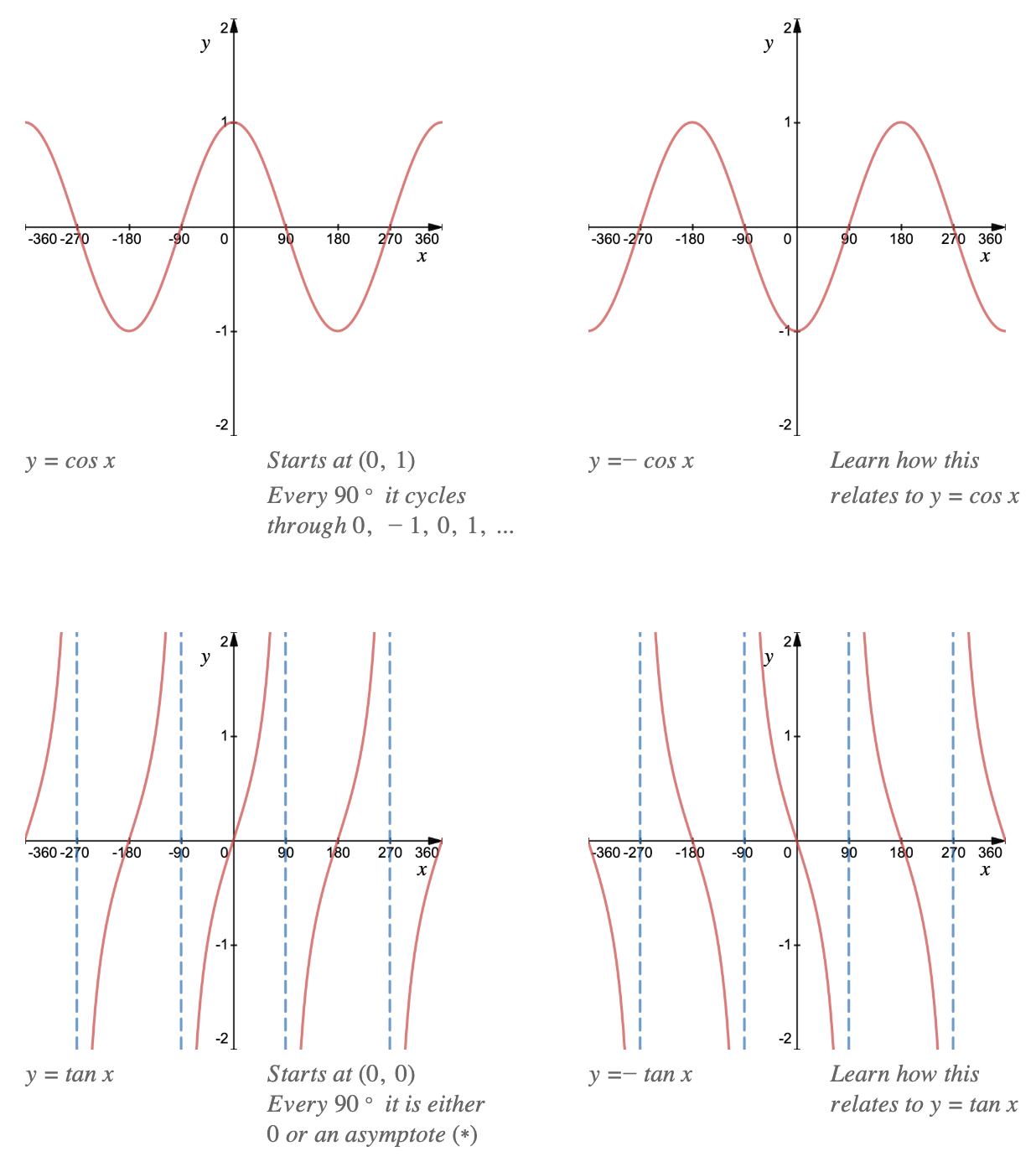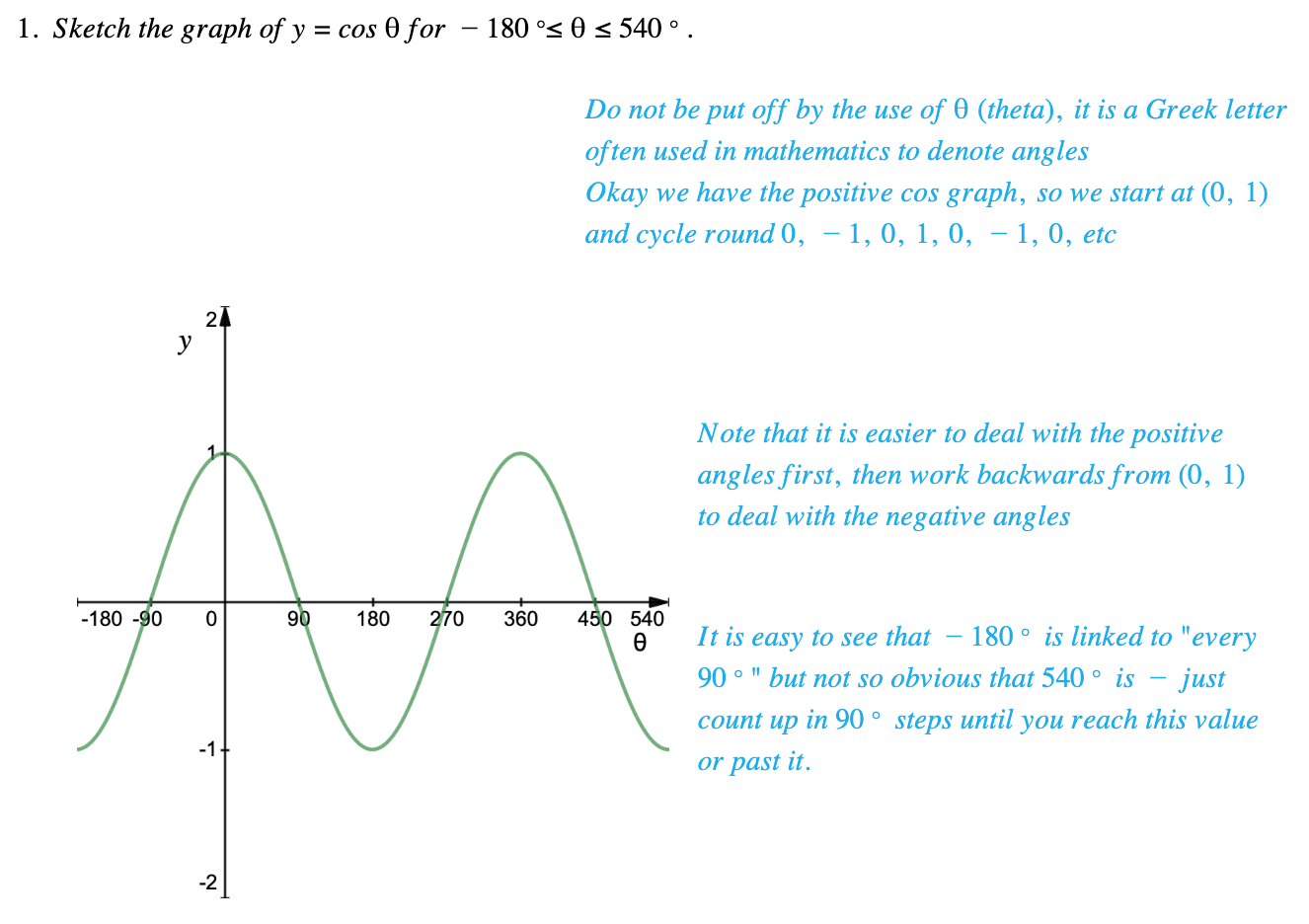# Edexcel IGCSE Maths 复习笔记 3.4.3 Drawing Graphs - Trig Graphs

Edexcel IGCSE Maths 复习笔记 3.4.3 Drawing Graphs - Trig Graphs

#### Why do we need to know what trigonometric graphs look like?

• Trigonometric Graphs are used in various applications of mathematics – for example, the oscillating nature can be used to model how a pendulum swings

#### Drawing graphs – trig graphs

• As with other graphs, being familiar with the general style of trigonometric graphs will help you sketch them quickly and you can then use them to find values or angles alongside your calculator
• All trigonometric graphs follow a pattern – a “starting point” and then “something happens every 90°”.• (*) An asymptote is a line that a graph gets ever closer to without ever crossing or touching it